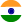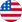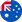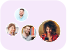24*7 support on WhatsAppChat NowProduct Edition:1st edition
Author: S. Selçuk Bayin
Book Name: Essentials of Mathematical Methods in Science and Engineering
Subject Name: Engineering

# Essentials of Mathematical Methods in Science and Engineering 1st edition Solutions

0 out of 5.0
43 reviews31 Students
have requested for homework help from this book

A complete introduction to the multidisciplinary applications of mathematical methods In order to work with varying levels of engineering and physics research, it is important to have a firm understanding of key mathematical concepts such as advanced calculus, differential equations, complex analysis, and introductory mathematical physics. Essentials of Mathematical Methods in Science and Engineering provides a comprehensive introduction to these methods under one cover, outlining basic mathematical skills while also encouraging students and practitioners to develop new, interdisciplinary approaches to their research. The book begins with core topics from various branches of mathematics such as limits, integrals, and inverse functions. Subsequent chapters delve into the analytical tools that are commonly used in scientific and engineering studies, including vector analysis, generalized coordinates, determinants and matrices, linear algebra, complex numbers, complex analysis, and Fourier series. The author provides an extensive chapter on probability theory with applications to statistical mechanics and thermodynamics that complements the following chapter on information theory, which contains coverage of Shannon's theory, decision theory, game theory, and quantum information theory. A comprehensive list of references facilitates further exploration of these topics. Throughout the book, numerous examples and exercises reinforce the presented concepts and techniques. In addition, the book is in a modular format, so each chapter covers its subject thoroughly and can be read independently. This structure affords flexibility for individualizing courses and teaching. Providing a solid foundation and overview of the various mathematical methods and applications in multidisciplinary research, Essentials of Mathematical Methods in Science and Engineering is an excellent text for courses in physics, science, mathematics, and engineering at the upper-undergraduate and graduate levels. It also serves as a useful reference for scientists and engineers who would like a practical review of mathematical methods.

5
22
4
9
3
13
2
4
1
0

0

## Students who viewed this book also checked out